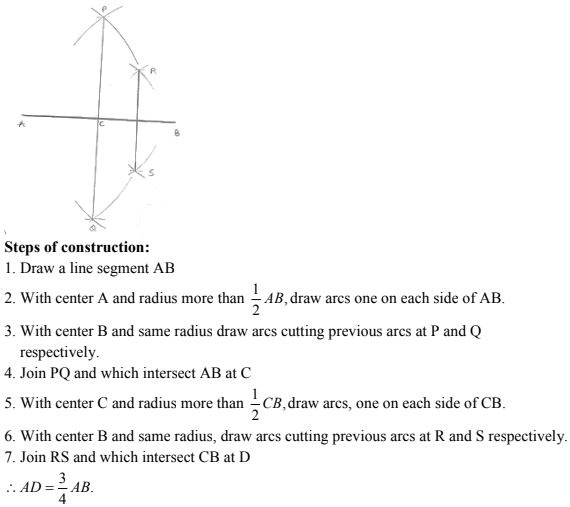# RD Sharma Solutions Chapter 11 Constructions Exercise 11.1 Class 10 Maths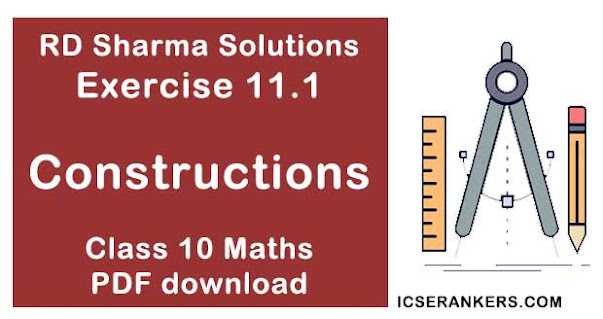Chapter Name RD Sharma Chapter 11 Constructions Book Name RD Sharma Mathematics for Class 10 Other Exercises Exercise 11.2Exercise 11.3 Related Study NCERT Solutions for Class 10 Maths

### Exercise 11.1 Solutions

1. Draw a line segment of length 8.6 cm. Bisect it and measure the length of each part.

Solution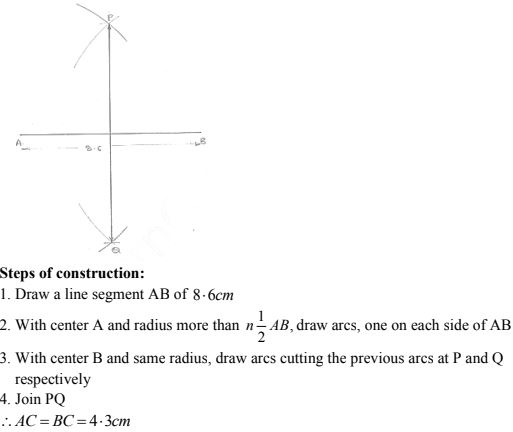2. Draw a line segment AB of length 5.8 cm . Draw the perpendicular bisector of this line segment.

Solution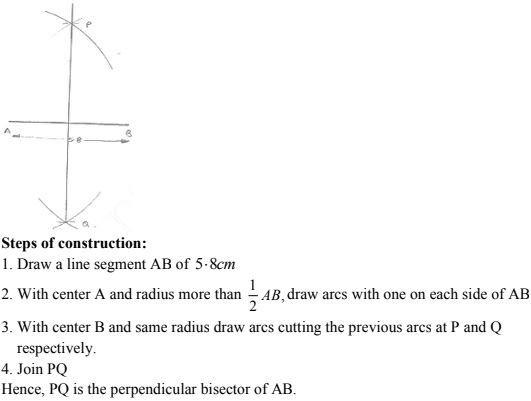3. Draw a circle with centre at point O and radius 5 cm. Draw its chord AB, draw the perpendicular bisector of line segment AB. Does it pass through the centre of the circle  ?

Solution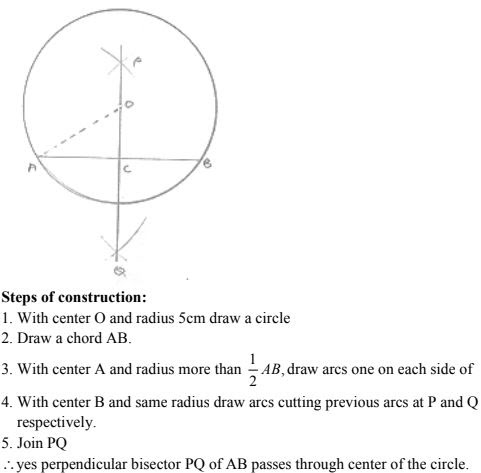4. Draw a circle with centre at point O. Draw its two chords AB and CD such that AB is not parallel to CD. Draw the perpendicular bisectors of AB and CD. At what point do they intersect ?

Solution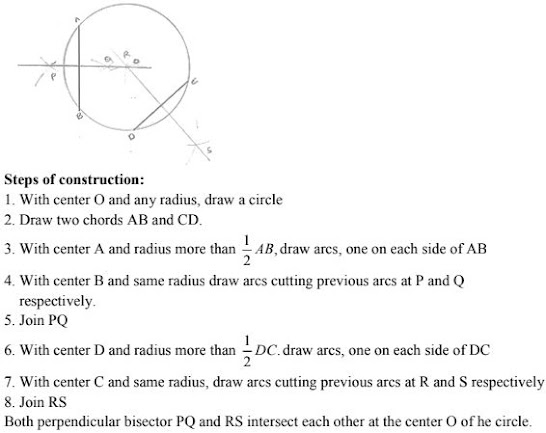5. Draw a line segment of length 10 cm and bisect it. Further bisect one of the equal parts and measure its length.

Solution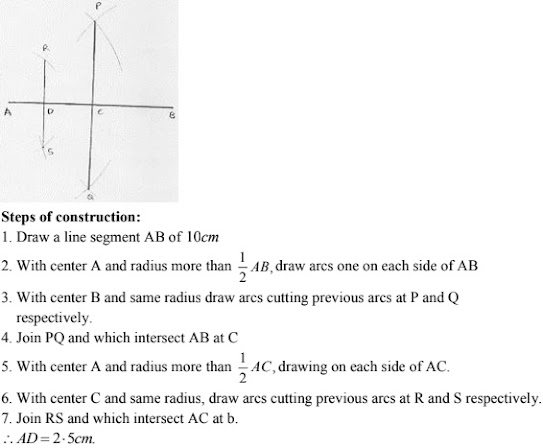6. Draw a line segment AB and bisect it. Bisect one of the equal parts to obtain a line segment of length 1/2 (AB).

Solution7. Draw a line segment AB and by ruler and compasses l obtain a line segment of length 3/4 (AB).

Solution